# Josephus problem | Set 1 (A O(n) Solution)

• Difficulty Level : Hard
• Last Updated : 08 Nov, 2021

In computer science and mathematics, the Josephus Problem (or Josephus permutation) is a theoretical problem. Following is the problem statement:

There are n people standing in a circle waiting to be executed. The counting out begins at some point in the circle and proceeds around the circle in a fixed direction. In each step, a certain number of people are skipped and the next person is executed. The elimination proceeds around the circle (which is becoming smaller and smaller as the executed people are removed), until only the last person remains, who is given freedom. Given the total number of persons n and a number k which indicates that k-1 persons are skipped and kth person is killed in circle. The task is to choose the place in the initial circle so that you are the last one remaining and so survive.
For example, if n = 5 and k = 2, then the safe position is 3. Firstly, the person at position 2 is killed, then person at position 4 is killed, then person at position 1 is killed. Finally, the person at position 5 is killed. So the person at position 3 survives.
If n = 7 and k = 3, then the safe position is 4. The persons at positions 3, 6, 2, 7, 5, 1 are killed in order, and person at position 4 survives.

Attention reader! Don’t stop learning now. Get hold of all the important DSA concepts with the DSA Self Paced Course at a student-friendly price and become industry ready.  To complete your preparation from learning a language to DS Algo and many more,  please refer Complete Interview Preparation Course.

In case you wish to attend live classes with experts, please refer DSA Live Classes for Working Professionals and Competitive Programming Live for Students.

The problem has following recursive structure.

```  josephus(n, k) = (josephus(n - 1, k) + k-1) % n + 1
josephus(1, k) = 1```

After the first person (kth from beginning) is killed, n-1 persons are left. So we call josephus(n – 1, k) to get the position with n-1 persons. But the position returned by josephus(n – 1, k) will consider the position starting from k%n + 1. So, we must make adjustments to the position returned by josephus(n – 1, k).

Following is simple recursive implementation of the Josephus problem. The implementation simply follows the recursive structure mentioned above.

## C++

 `#include ``using` `namespace` `std;` `int` `josephus(``int` `n, ``int` `k)``{``    ``if` `(n == 1)``        ``return` `1;``    ``else``        ``/* The position returned by josephus(n - 1, k)``        ``is adjusted because the recursive call``        ``josephus(n - 1, k) considers the``        ``original position k % n + 1 as position 1 */``        ``return` `(josephus(n - 1, k) + k - 1) % n + 1;``}` `// Driver Program to test above function``int` `main()``{``    ``int` `n = 14;``    ``int` `k = 2;``    ``cout << ``"The chosen place is "` `<< josephus(n, k);``    ``return` `0;``}` `// This code is contributed by shubhamsingh10`

## C

 `#include ` `int` `josephus(``int` `n, ``int` `k)``{``    ``if` `(n == 1)``        ``return` `1;``    ``else``        ``/* The position returned by josephus(n - 1, k) is``           ``adjusted because the recursive call josephus(n -``           ``1, k) considers the original position``           ``k%n + 1 as position 1 */``        ``return` `(josephus(n - 1, k) + k - 1) % n + 1;``}` `// Driver Program to test above function``int` `main()``{``    ``int` `n = 14;``    ``int` `k = 2;``    ``printf``(``"The chosen place is %d"``, josephus(n, k));``    ``return` `0;``}`

## Java

 `// Java code for Josephus Problem``import` `java.io.*;` `class` `GFG {` `    ``static` `int` `josephus(``int` `n, ``int` `k)``    ``{``        ``if` `(n == ``1``)``            ``return` `1``;``        ``else``            ``/* The position returned by josephus(n - 1, k)``            ``is adjusted because the recursive call``            ``josephus(n - 1, k) considers the original``            ``position k%n + 1 as position 1 */``            ``return` `(josephus(n - ``1``, k) + k - ``1``) % n + ``1``;``    ``}` `    ``// Driver Program to test above function``    ``public` `static` `void` `main(String[] args)``    ``{``        ``int` `n = ``14``;``        ``int` `k = ``2``;``        ``System.out.println(``"The chosen place is "``                           ``+ josephus(n, k));``    ``}``}` `// This code is contributed by Prerna Saini`

## Python3

 `# Python code for Josephus Problem`  `def` `josephus(n, k):` `    ``if` `(n ``=``=` `1``):``        ``return` `1``    ``else``:` `        ``# The position returned by``        ``# josephus(n - 1, k) is adjusted``        ``# because the recursive call``        ``# josephus(n - 1, k) considers``        ``# the original position``        ``# k%n + 1 as position 1``        ``return` `(josephus(n ``-` `1``, k) ``+` `k``-``1``) ``%` `n ``+` `1` `# Driver Program to test above function`  `n ``=` `14``k ``=` `2` `print``(``"The chosen place is "``, josephus(n, k))` `# This code is contributed by``# Sumit Sadhakar`

## C#

 `// C# code for Josephus Problem``using` `System;` `class` `GFG {` `    ``static` `int` `josephus(``int` `n, ``int` `k)``    ``{``        ``if` `(n == 1)``            ``return` `1;``        ``else``            ``/* The position returned``            ``by josephus(n - 1, k) is``            ``adjusted because the``            ``recursive call josephus(n``            ``- 1, k) considers the``            ``original position k%n + 1``            ``as position 1 */``            ``return` `(josephus(n - 1, k) + k - 1) % n + 1;``    ``}` `    ``// Driver Program to test above``    ``// function``    ``public` `static` `void` `Main()``    ``{``        ``int` `n = 14;``        ``int` `k = 2;``        ``Console.WriteLine(``"The chosen "``                          ``+ ``"place is "` `+ josephus(n, k));``    ``}``}` `// This code is contributed by anuj_67.`

## PHP

 ``

## Javascript

 ``
Output
`The chosen place is 13`

Time Complexity: O(n)

Auxiliary Space: O(n)

### Another Approach using List:

The simple approach is to create a list and add all values from 1 to n in it. Create a recursive function that takes list, start (position at which counting will start) and k ( number of person to be skipped) as argument. If size of list is one i.e. only one person left then return this position. Otherwise, start counting k person in clockwise direction from starting position and remove the person at kth position. Now the person at kth position is removed and now counting will start from this position. This process continues till only one person left.

```pseudo-code :

Josephus( list , start , k){
if list.size = 1
return list
start = (start + k) % list.size
list.remove( start )
return Josephus( list, start, k)
}```

C++ Code

## C++

 `#include ` `using` `namespace` `std;` `void` `Josh(vector<``int``> person, ``int` `k, ``int` `index)``{``    ``// Base case , when only one person is left``    ``if` `(person.size() == 1) {``        ``cout << person << endl;``        ``return``;``    ``}` `    ``// find the index of first person which will die``    ``index = ((index + k) % person.size());` `    ``// remove the first person which is going to be killed``    ``person.erase(person.begin() + index);` `    ``// recursive call for n-1 persons``    ``Josh(person, k, index);``}` `int` `main()``{``    ``int` `n = 14; ``// specific n and k  values for original``                ``// josephus problem``    ``int` `k = 2;``    ``k--; ``// (k-1)th person will be killed``    ``int` `index``        ``= 0; ``// The index where the person which will die` `    ``vector<``int``> person;``    ``// fill the person vector``    ``for` `(``int` `i = 1; i <= n; i++) {``        ``person.push_back(i);``    ``}` `    ``Josh(person, k, index);``}`

## C#

 `using` `System;``using` `System.Collections.Generic;``class` `GFG {``    ` `    ``static` `void` `Josh(List<``int``> person, ``int` `k, ``int` `index)``    ``{``        ``// Base case , when only one person is left``        ``if` `(person.Count == 1) {``            ``Console.WriteLine(person);``            ``return``;``        ``}``     ` `        ``// find the index of first person which will die``        ``index = ((index + k) % person.Count);``     ` `        ``// remove the first person which is going to be killed``        ``person.RemoveAt(index);``     ` `        ``// recursive call for n-1 persons``        ``Josh(person, k, index);``    ``}` `  ``// Driver code``  ``static` `void` `Main()``  ``{``    ``int` `n = 14; ``// specific n and k  values for original``                ``// josephus problem``    ``int` `k = 2;``    ``k--; ``// (k-1)th person will be killed``    ``int` `index``        ``= 0; ``// The index where the person which will die`` ` `    ``List<``int``> person = ``new` `List<``int``>();``    ``// fill the person vector``    ``for` `(``int` `i = 1; i <= n; i++) {``        ``person.Add(i);``    ``}`` ` `    ``Josh(person, k, index);``  ``}``}` `// This code is contributd by divyesh072019.`
Output

`13`

Time Complexity: O(n)

Example

```Input : n = 5,  k = 2
output : 3```

Explanation :

Add all values from 1 to n in the list. We will call the recursive function with start = 0 and k = 1 (0-indexing)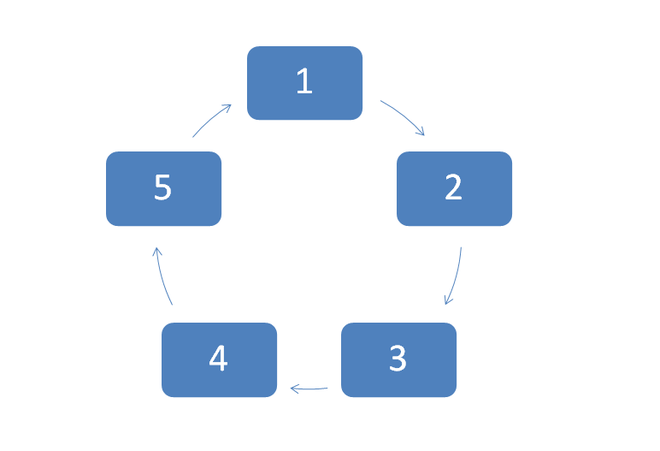Now the element at 1-index (person number 2) will be killed. And it is removed from list. The new counting will begins from 1-index, person at 1-index killed so now person at 2-index (person number 3) comes to 1-index and counting starts from here now.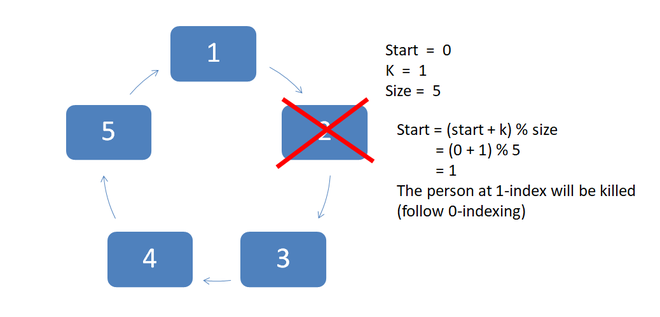Now we have 4 person, counting start from 1-index (person number 3) and the person at kth (2-index ) position will be killed.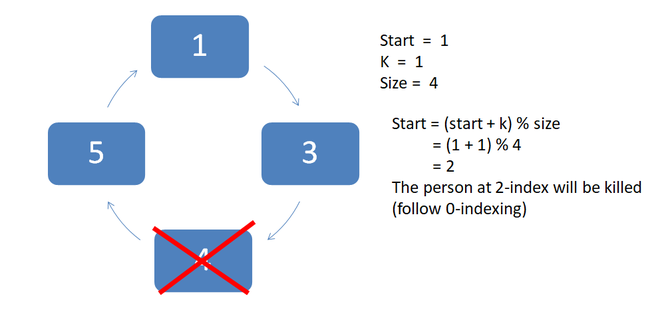The person at 2-index (person number 4) killed so now we have 3 person left and the person (person number 5) at 3-index shifted to 2-index. And counting starts from here.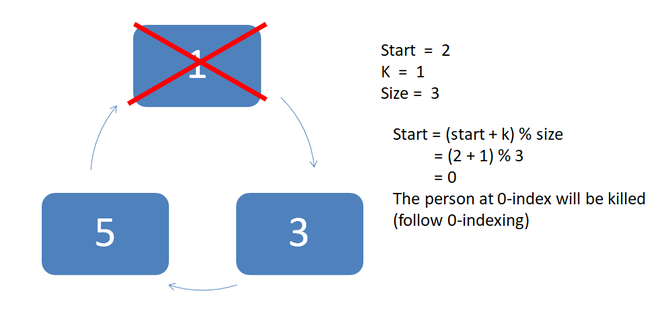The person at 0-index killed and we have now two person left in the circle. And the person at 1-index shifted to 0-index i.e. person number 3.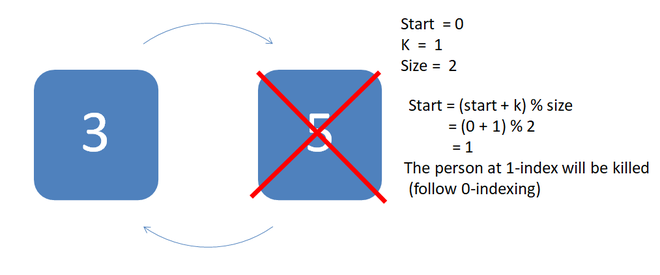Final counting done and person at 1-index killed and only person who is left is at position 3.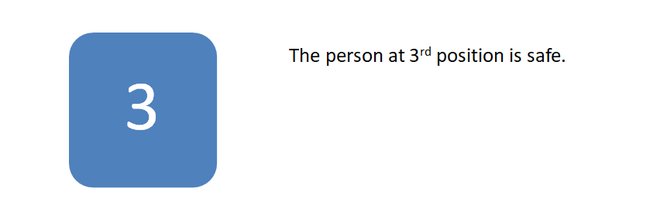This is a solution to the Josephus problem in C++.

For n = 47 and k = 5.

## C++

 `#include ``using` `namespace` `std;` `int` `Josephus(``int``, ``int``);` `int` `main()``{``    ``int` `n, k;``    ``cin >> n >> k;``    ``cout << Josephus(n, k);``    ``return` `0;``}` `int` `Josephus(``int` `n, ``int` `k)``{``    ``k--;``    ``int` `arr[n];``    ``for` `(``int` `i = 0; i < n; i++) {``        ``arr[i] = 1; ``// Makes all the 'n' people alive by``                    ``// assigning them value = 1``    ``}``    ``int` `cnt = 0, cut = 0,``        ``num = 1; ``// Cut = 0 gives the sword to 1st person.``    ``while` `(``        ``cnt``        ``< (n - 1)) ``// Loop continues till n-1 person dies.``    ``{``        ``while` `(num <= k) ``// Checks next (kth) alive persons.``        ``{``            ``cut++;``            ``cut = cut % n; ``// Checks and resolves overflow``                           ``// of Index.``            ``if` `(arr[cut] == 1) {``                ``num++; ``// Updates the number of persons``                       ``// alive.``            ``}``        ``}``        ``num = 1; ``// refreshes value to 1 for next use.``        ``arr[cut]``            ``= 0; ``// Kills the person at position of 'cut'``        ``cnt++; ``// Updates the no. of killed persons.``        ``cut++;``        ``cut = cut``              ``% n; ``// Checks and resolves overflow of Index.``        ``while` `(arr[cut]``               ``== 0) ``// Checks the next alive person the``                     ``// sword is to be given.``        ``{``            ``cut++;``            ``cut = cut % n; ``// Checks and resolves overflow``                           ``// of Index.``        ``}``    ``}``    ``return` `cut + 1; ``// Output is the position of the last``                    ``// man alive(Index + 1);``}` `/********************THIS CODE IS PRESENTED BY SHISHANK`` ``* RAWAT**************************/`

If you guys liked it, please comment and help me with further improvements.

Output
`The safe position : 4`

Josephus problem | Set 2 (A Simple Solution when k = 2)